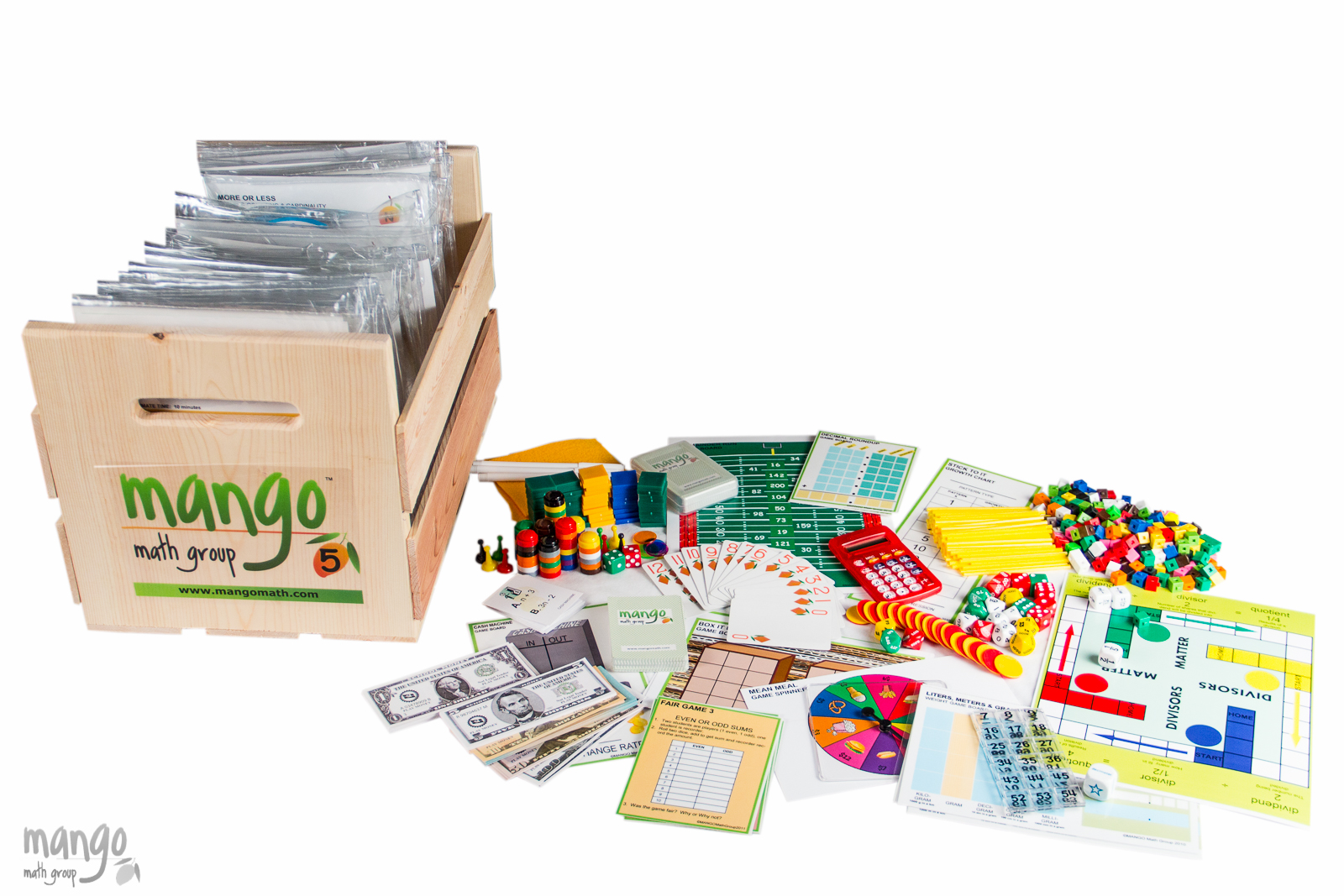#### Order Notice

As the economy opens back up, many of our suppliers are experiencing shortages of parts and raw materials, resulting in delays in shipments. As a result, we anticipate that it may take up to 2-4 weeks from the date we receive a purchase order for us to fulfill and ship any order for our products. We apologize for any inconvenience.

# Fifth Grade Deluxe Math Kit

Our Strong Evidence Based Fifth Grade Deluxe Math Kit contains 20 separate skill building math lessons to supplement the fifth grade math curriculum.

\$ 419.00 USD##### Product Details

MANGO Math's Fifth Grade Deluxe Math Kit contains 20 separate skill building math lessons.

Each individual lesson offers visual and kinetic representation of math concepts that develop strong math knowledge, like:

• developing understanding of multiplication of fractions
• expanding division
• expanding place value into decimals and developing fluency

MANGO Math's Strong Evidence Based Kits come with individually packaged, Grab-n-Go lessons that include instructions and reusable materials, pre-packaged in a resealable pouch. These are contained in an easy-to-carry crate that can be used year after year.

Grade-level math kits, set up in math center format, can meet the needs of 30+ students at a time. Lessons within a kit can be used by up to 4 students.

Every lesson is based on a game or activity that fosters curiosity, discussion and growth, through cooperation, collaboration and creative thinking.

Each MANGO Math grade level math kit is research-based and adheres to Common Core, TEKS and NCTM math standards.

Kits are great for use in regular classrooms, resource rooms, after school programs, STEM programs, libraries, math centers, summer camps, learning cooperatives, math clubs, community centers, and at home.

#### All the supplies below are included with its specific lesson in the kit:

• spinner
• 6-sided dice
• 10-sided dice
• 10s dice
• 100s dice
• 1000s dice
• bi-colored counters
• transparent counters
• centimeter cubes
• stacking counters
• colored tiles
• counting sticks
• play money: dollars and coins
• anglegs
• protractors
• calculators
• MANGO Math playing cards
• numbered tiles
• game pawns
• plastic resealable pouch
• game boards
• laminated writing boards
• dry erase marker
• curriculum guide

## Fifth Grade Lessons & Standards

Liters, Meters, and Grams – Measurement: Convert among different-sized standard measurement units within a given measurement system (convert 5 cm to.05 m)

Sugar Heights – Measurement: Measure volumes by counting unit cubes, using cubic cm. Relate volume to the operation of multiplication and addition and solve real world problems.

Cash Machine – Algebraic Thinking: Generate numerical patterns using two given rules.  Identify apparent relationships between terms.

Counting Cards – Algebraic Thinking: Use parentheses, brackets, or braces to numerical expressions and evaluate expressions with these symbols.

Stick To It – Algebraic Thinking: Generate numerical patterns using two given rules.  Identify relationships between rules.

Box It Up – Number Operations – Fraction: Add fractions with unlike denominators by replacing given fractions with equivalent fractions.

Close Call – Number Operations– Base 10: Fluently multiply multi-digit whole numbers using strategies placed on place value understanding.

Decimal Roundup – Number Operations – Base 10: Use place value understanding to round decimals to any place.

Ditto –Number Operations – Fraction: (sample lesson) Replace given fractions with equivalent fractions in such a way as to produce an equivalent sum or difference of fractions.

Divisors Matter – Number Operations – Base 10: Apply and extend previous understanding of division to divide unit fractions by whole numbers and whole numbers by unit fractions.

Golden Ticket – Number Operation – Fraction: Solve real world problems involving multiplication of fractions & mixed numbers by using fractional models.

Great Divide – Number Operation – Base 10: Find whole-number quotients of whole numbers using strategies based on properties of operations.

LCM/LCD– Number Operation – Base 10: Add and subtract fractions with unlike denominators by replacing given fractions with equivalent fractions.

Remainder Run – Number Operations – Base 10: Find whole-number quotients of whole numbers with up to four-digit dividends and two-digit divisors, using strategies.

Two Point Five – Number Operations – Base 10: Explain patterns in the number of zeros of the product when multiplying a number by powers of 10, and explain patterns in the placement of decimal point.

Nine Men Morris – Problem Solving: Mathematical Practices:  Reason abstractly, make sense of problems and persevere, Look for and express regularity in repeated reasoning.

Square Deal – Problem Solving: Mathematical Practices:  Make sense of problems and persevere in solving them.  Look for and express regularity in repeated reasoning.

Treasure Hunt – Geometry: Graph points on the coordinate plane to solve real-world and mathematical problems.

Fair Game – Odds & Order: Make a line plot to display a data set of measurement in fractions of units.  Use fractions to solve problems using line plot information.

Mean Meal – Odds &Order: Make a line plot to display a data set of measurements. Use operations to solve problems involving information present in line plot.

Width
12
in
Height
12
in
Length
18
in
Weight
20
lb
{% if context == "cart" or context == "checkout" %} {% endif %}